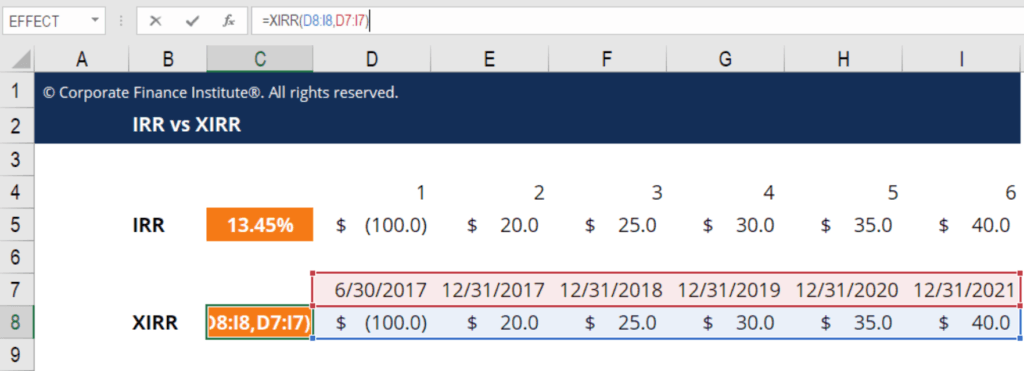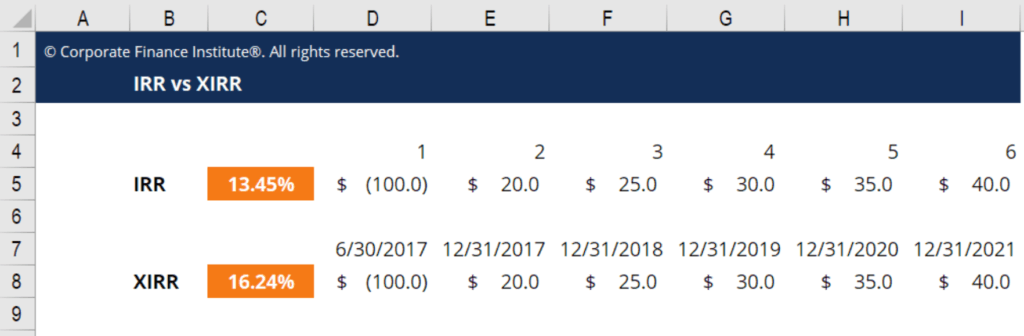# XIRR vs IRR

Why you should always use XIRR instead of IRR in financial modeling

## What is XIRR vs IRR in Excel?

In financial modeling and valuation, it’s critical to understand why to use XIRR vs IRR.  Using the simple =IRR function in Excel can be dangerous, as it assumes all the time periods in a series of cash flows are equal.  This is frequently not the case, especially if you have an initial investment up front, which is almost never on December 31.

XIRR gives you the flexibility to assign specific dates to each individual cash flow, making it a much more accurate calculation.### What is Internal Rate of Return (IRR)?

The Internal Rate of Return is the discount rate which sets the Net Present Value (NPV) of all future cash flow of an investment to zero.  If the NPV of an investment is zero it doesn’t mean it’s a good or bad investment, it just means you will earn the IRR (discount rate) as your rate of return.

### What is IRR in Excel?

If you use the =IRR() formula in Excel you are using equal time periods between each cell (cash flow).  This makes it challenging when you expect to enter an investment in the middle of a year.  For this reason, (as outlined below) always use XIRR instead.

### What is XIRR in Excel?

If you use the =XIRR() formula in Excel you have complete flexibility over the time periods of the cash flows.  In order to do this, enter two series in your formula:

• The series of cash flows
• The corresponding dates of each of the cash flows

### Example of XIRR vs IRR calculation

Below is an example of regular IRR versus XIRR with a series of six cash flows.  With regular IRR it assumes all cash flows are on Dec 31, but with XIRR we can tell Excel that the first cash flow is in the middle of the year.  This has a meaningful impact on the actual internal rate of return.As you can see in the result below, using XIRR vs IRR produces 16.25% compared to 13.45%, which is a material difference!